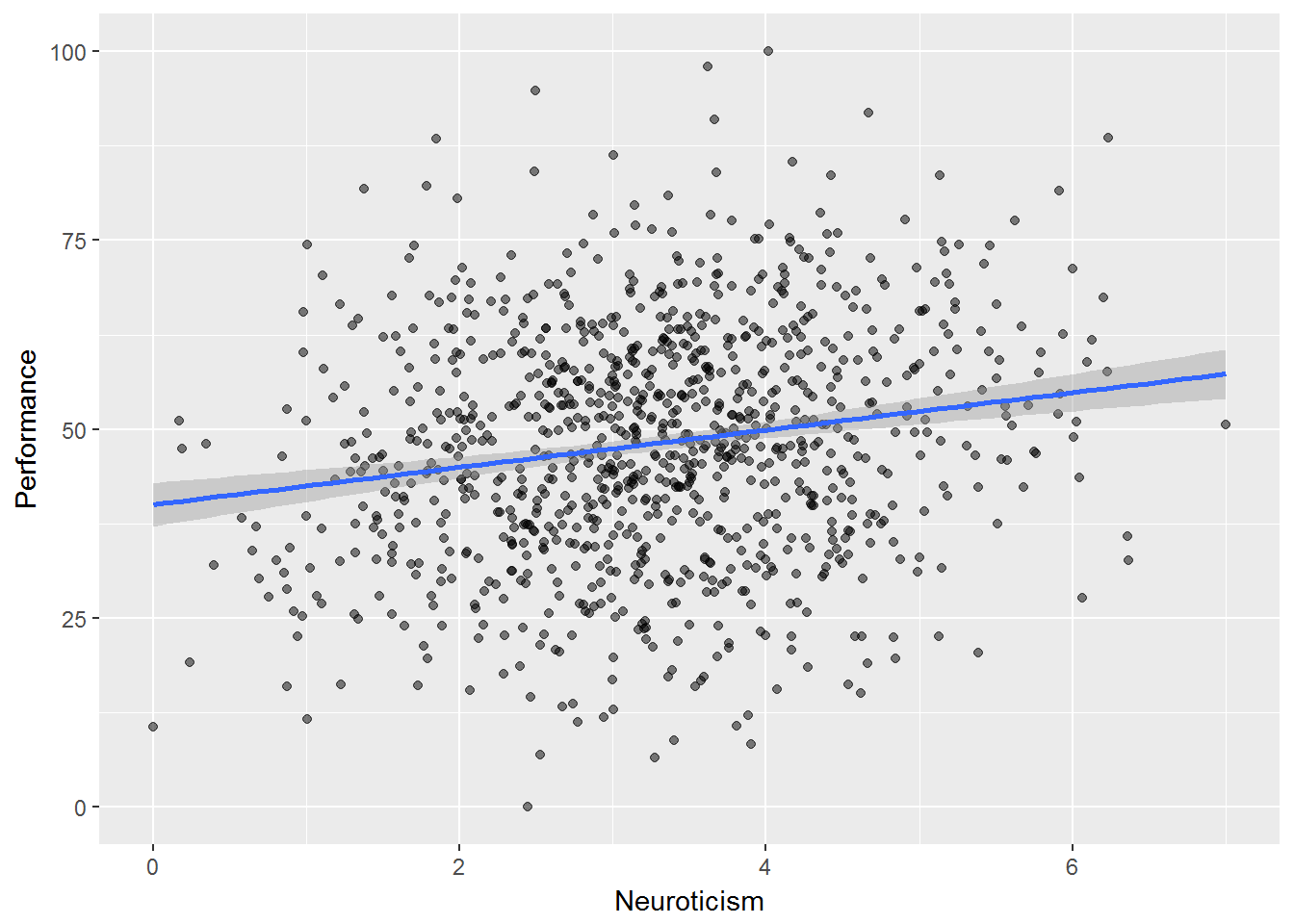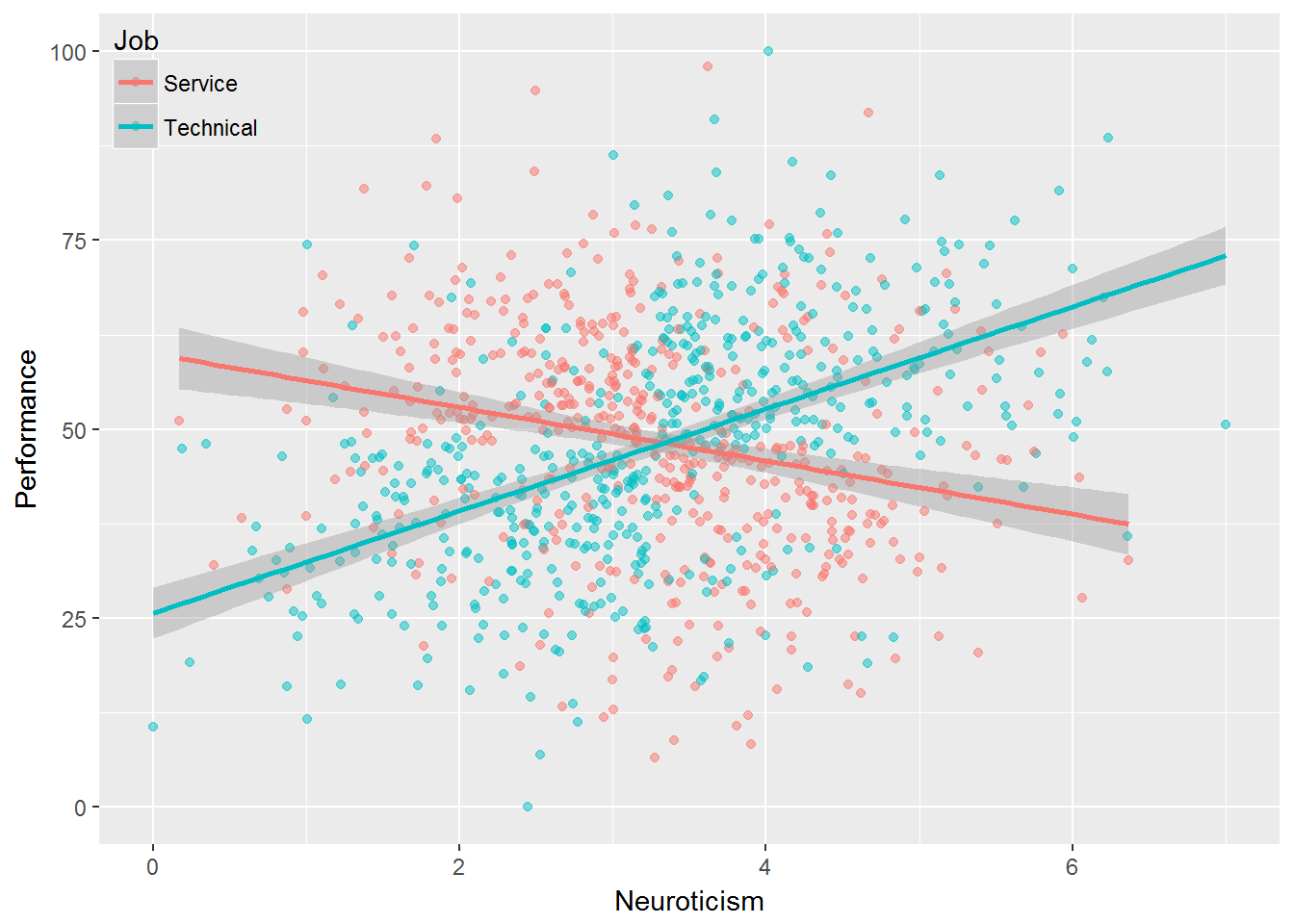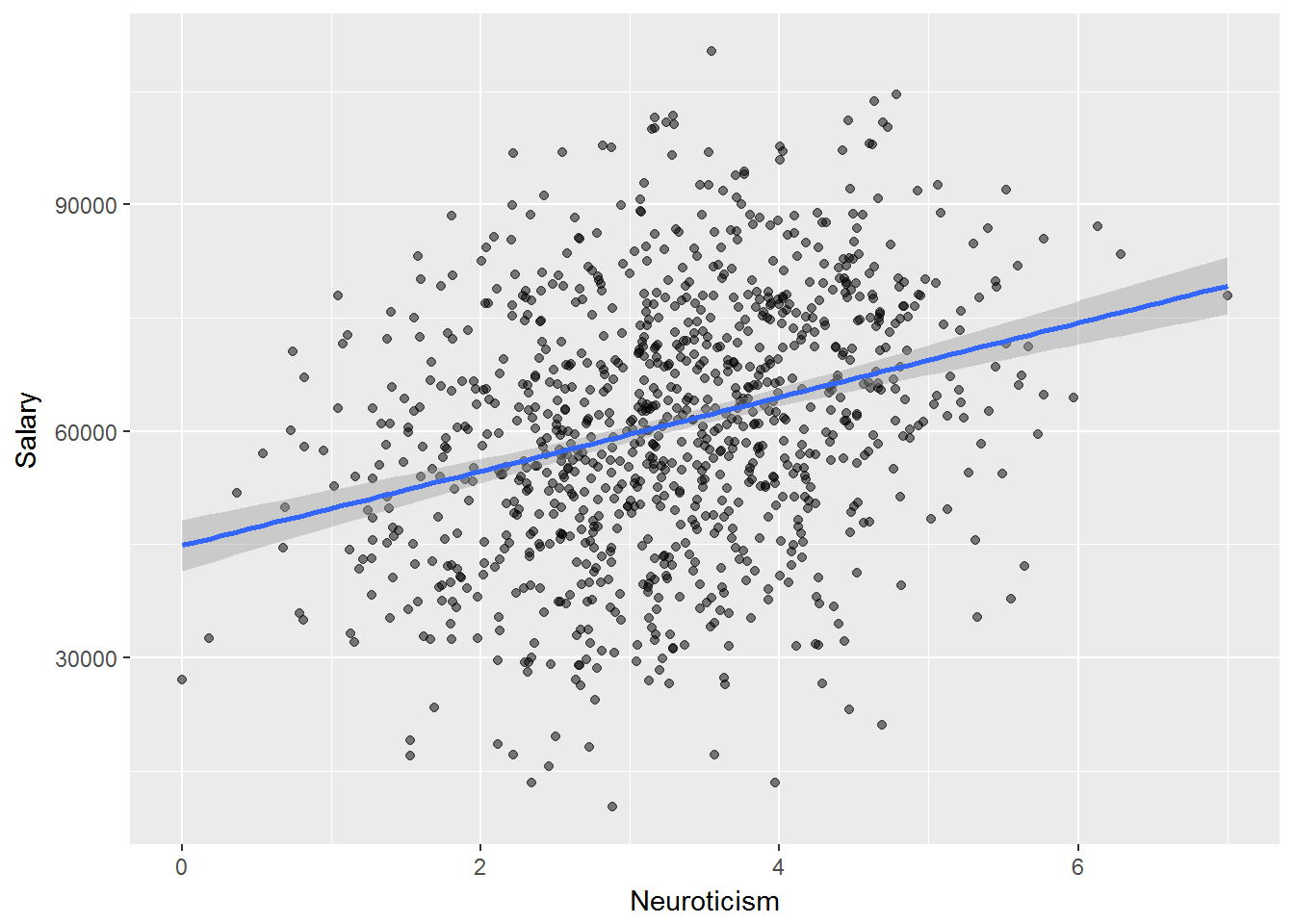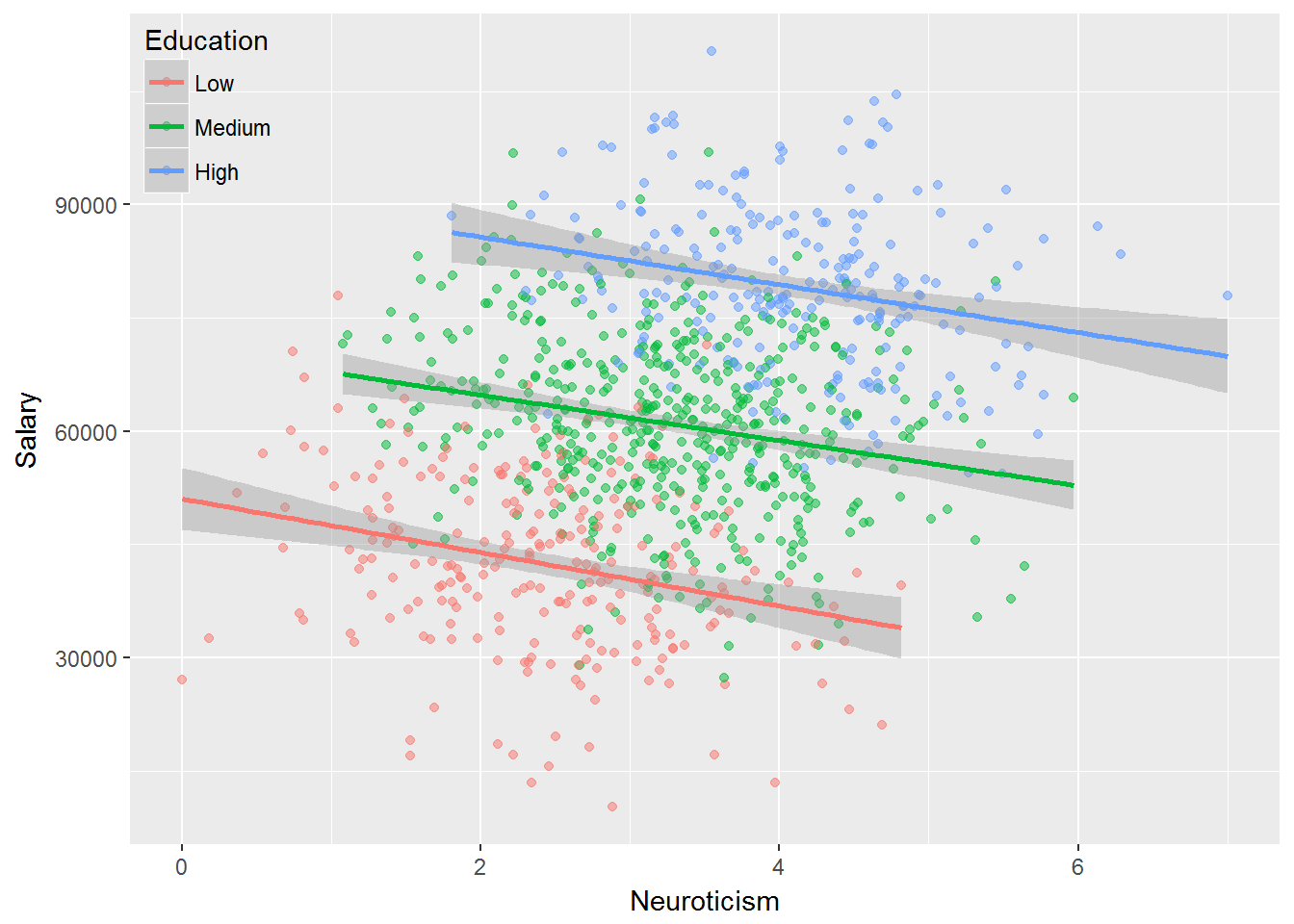library(dplyr)
##
## Attaching package: 'dplyr'
## The following objects are masked from 'package:stats':
##
##     filter, lag
## The following objects are masked from 'package:base':
##
##     intersect, setdiff, setequal, union
library(ggplot2)
library(scales)
alpha = 0.5
set.seed(123)
n = 1000

Neuroticism = rnorm(n)
Performance = rnorm(n) + Neuroticism * 0.1

Performance = rescale(Performance, to = c(0, 100))
# summary(Performance)
Neuroticism = rescale(Neuroticism, to = c(0, 7))
# summary(Neuroticism)

data <- data.frame(
Performance,
Neuroticism
)

options = c("Technical","Service")
technical =
(data$Performance > mean(data$Performance) &
data$Neuroticism > mean(data$Neuroticism)) |
(data$Performance < mean(data$Performance) &
data$Neuroticism < mean(data$Neuroticism))

data$Job[technical] <- sample(options, sum(technical), T, c(0.6, 0.2)) data$Job[is.na(data$Job)] <- sample(options, sum(is.na(data$Job)), T, c(0.2, 0.8))

p <- data %>% ggplot(aes(Neuroticism, Performance))
p + geom_point(alpha = alpha) + geom_smooth(method = 'lm')p +
geom_point(aes(col = Job), alpha = alpha) +
geom_smooth(aes(col = Job), method = 'lm') +
theme(legend.background = element_rect(fill = "transparent"),
legend.justification = c(0, 1),
legend.position = c(0, 1))set.seed(123)
n = 1000

Education = rbinom(n, 2, 0.5)
Neuroticism = rnorm(n) + Education
Salary = Education * 2 + rnorm(n) - Neuroticism * 0.3

Salary = sample(10000:11000,1) + rescale(Salary, to = c(0, 100000))
# summary(Salary)
Neuroticism = rescale(Neuroticism, to = c(0, 7))
# summary(Neuroticism)
Education = factor(Education, labels = c("Low", "Medium", "High"))

data <- data.frame(
Salary,
Neuroticism,
Education
)

p <- data %>% ggplot(aes(Neuroticism, Salary))
p + geom_point(alpha = alpha) + geom_smooth(method = 'lm')p +
geom_point(aes(col = Education), alpha = alpha) +
geom_smooth(aes(col = Education), method = 'lm') +
theme(legend.background = element_rect(fill = "transparent"),
legend.justification = c(0, 1),
legend.position = c(0, 1))